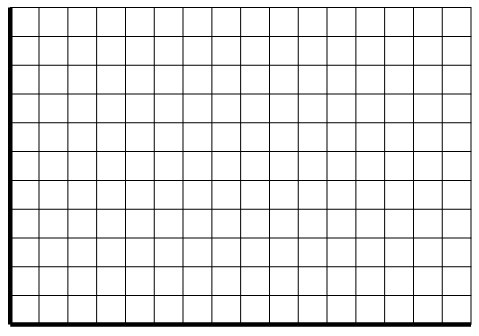# LINR 1 | Lesson 2 | Practice (Graphs of Linear Situations)Practice

## Linear Situation

A catering company charges $15.00 per guest and a flat rate of$100.00 to cater a luncheon.

## Table

1. Complete the table below to represent the cost for up to 80 guests.  Follow these steps:
• Determine the appropriate $$x$$values needed to complete a table that represents the cost for up to 80 guests.
• What is the starting $$x$$-value?  This value should be in the first row of the table.
• What $$x$$value should be in the last row of the table?
• By how much should the $$x$$-values increase from one line to the next?## Graph

2. Use the values in your table and a coordinate plane like the one shown below to graph this situation.

• Label each axis.
• Let the intersection of the axes represent the origin $$(0,0)$$.
• Determine the scale for each axis . Be sure that the graph shows up to at least 80 guests.## Equation

2. Determine the slope and the $$y$$-intercept for this situation.

Slope:

$$y$$-intercept:

3. Define two variables to use in this situation.

Let _ =

Let _ =

4. Write an equation to represent the total cost to cater a luncheon for any number of guests.

Check solutions here.Explore!